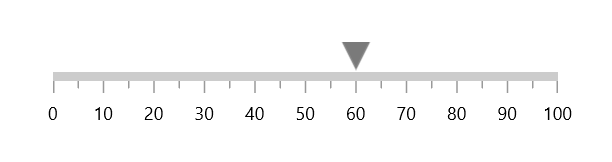# Shape Pointer in WinUI Linear Gauge

26 May 20218 minutes to read

Indicate current values by using the different types of shape pointers. You can change the shape type using the `ShapeType` property.

``````<gauge:SfLinearGauge>
<gauge:SfLinearGauge.Axis>
<gauge:LinearAxis>
<gauge:LinearAxis.MarkerPointers>
<gauge:ShapePointer Value="60" />
</gauge:LinearAxis.MarkerPointers>
</gauge:LinearAxis>
</gauge:SfLinearGauge.Axis>
</gauge:SfLinearGauge>``````
``````SfLinearGauge sfLinearGauge = new SfLinearGauge();

ShapePointer shapePointer = new ShapePointer();
shapePointer.Value = 60;
sfLinearGauge.Axis.MarkerPointers.Add(shapePointer);

this.Content = sfLinearGauge;``````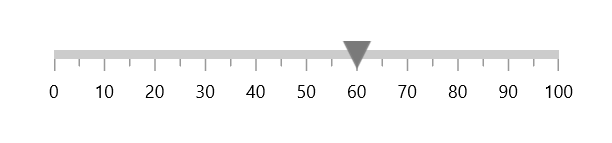Gauge supports the following types of shape pointer:

• Circle
• Diamond
• Inverted triangle
• Triangle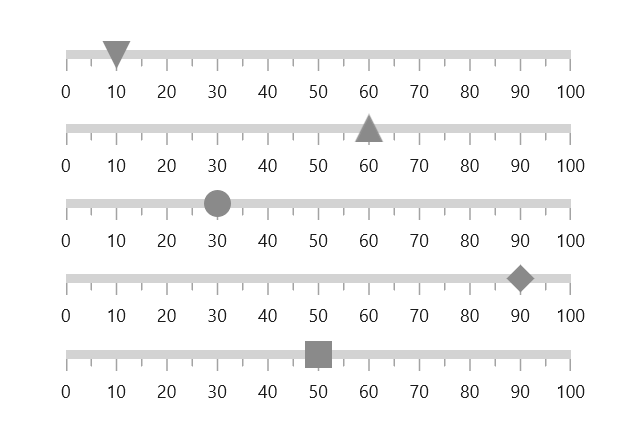## Custom shape pointer

You can add customized shapes to denote the current pointer value instead of in-built shape. It can be achieved by providing required shape in `ShapeTemplate` property.

``````<Page.Resources>
<DataTemplate x:Key="CustomShapePointer">
<Grid>
<Rectangle Fill="{Binding Fill}"
Stroke="{Binding Stroke}"
StrokeThickness="{Binding SrokeThickness}"
Width="{Binding ShapeHeight}"
Height="{Binding ShapeHeight}"
RadiusX="3"
RadiusY="3" />
</Grid>
</DataTemplate>
</Page.Resources>

<gauge:SfLinearGauge>
<gauge:SfLinearGauge.Axis>
<gauge:LinearAxis>
<gauge:LinearAxis.MarkerPointers>
<gauge:ShapePointer Value="60"
ShapeTemplate="{StaticResource CustomShapePointer}"/>
</gauge:LinearAxis.MarkerPointers>
</gauge:LinearAxis>
</gauge:SfLinearGauge.Axis>
</gauge:SfLinearGauge>``````
``````SfLinearGauge sfLinearGauge = new SfLinearGauge();

ShapePointer shapePointer = new ShapePointer();
shapePointer.Value = 60;
shapePointer.ShapeTemplate = this.Resources["CustomShapePointer"] as DataTemplate;
sfLinearGauge.Axis.MarkerPointers.Add(shapePointer);

this.Content = sfLinearGauge;``````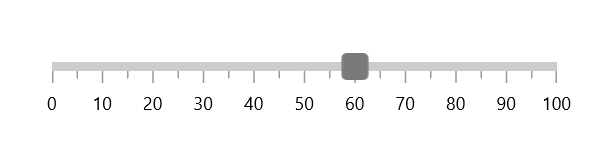## Shape customization

The shape pointer can be customized using the following properties:

``````<gauge:SfLinearGauge>
<gauge:SfLinearGauge.Axis>
<gauge:LinearAxis>
<gauge:LinearAxis.MarkerPointers>
<gauge:ShapePointer Value="60"
ShapeHeight="30"
ShapeWidth="30"
Fill="LightBlue"
Stroke="Black"
StrokeThickness="3"
ShapeType="Circle" />
</gauge:LinearAxis.MarkerPointers>
</gauge:LinearAxis>
</gauge:SfLinearGauge.Axis>
</gauge:SfLinearGauge>``````
``````SfLinearGauge sfLinearGauge = new SfLinearGauge();

ShapePointer shapePointer = new ShapePointer();
shapePointer.Value = 60;
shapePointer.ShapeHeight = 30;
shapePointer.ShapeWidth = 30;
shapePointer.Fill = new SolidColorBrush(Colors.LightBlue);
shapePointer.Stroke = new SolidColorBrush(Colors.Black);
shapePointer.StrokeThickness = 3;
shapePointer.ShapeType = GaugeShapeType.Circle;
sfLinearGauge.Axis.MarkerPointers.Add(shapePointer);

this.Content = sfLinearGauge;``````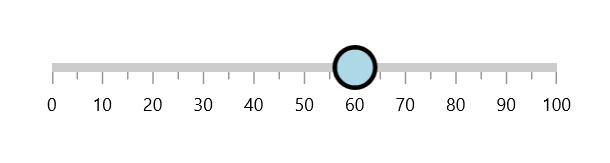## Shape position customization

The shape pointer can be moved near or far from its actual position in X or Y direction using the `OffsetPoint` property.

To move the pointer inside of the axis, provide positive values.

To move the pointer outside of the axis, provide negative values.

``````<gauge:SfLinearGauge>
<gauge:SfLinearGauge.Axis>
<gauge:LinearAxis>
<gauge:LinearAxis.MarkerPointers>
<gauge:ShapePointer Value="60"
OffsetPoint="0,-25"/>
</gauge:LinearAxis.MarkerPointers>
</gauge:LinearAxis>
</gauge:SfLinearGauge.Axis>
</gauge:SfLinearGauge>``````
``````SfLinearGauge sfLinearGauge = new SfLinearGauge();

ShapePointer shapePointer = new ShapePointer();
shapePointer.Value = 60;
shapePointer.OffsetPoint = new Point(0, -25);
sfLinearGauge.Axis.MarkerPointers.Add(shapePointer);

this.Content = sfLinearGauge;``````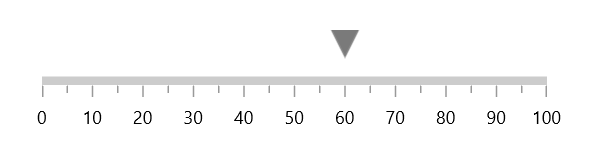### Shape pointer alignment

You can change the horizontal or vertical position of the shape pointer either start, end or center by using the `HorizontalAnchor` and `VerticalAnchor` properties. The default value of `HorizontalAnchor` and `VerticalAnchor` properties are center.

``````<gauge:SfLinearGauge>
<gauge:SfLinearGauge.Axis>
<gauge:LinearAxis>
<gauge:LinearAxis.MarkerPointers>
<gauge:ShapePointer Value="60"
VerticalAnchor="End"
OffsetPoint="0,-5" />
</gauge:LinearAxis.MarkerPointers>
</gauge:LinearAxis>
</gauge:SfLinearGauge.Axis>
</gauge:SfLinearGauge>``````
``````SfLinearGauge sfLinearGauge = new SfLinearGauge();

ShapePointer shapePointer = new ShapePointer();
shapePointer.Value = 60;
shapePointer.VerticalAnchor = GaugeAnchor.End;
shapePointer.OffsetPoint = new Point(0, -5);
sfLinearGauge.Axis.MarkerPointers.Add(shapePointer);

this.Content = sfLinearGauge;``````